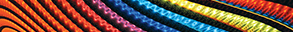Journal metrics
Acceptance rate13%
Submission to final decision50 days
Acceptance to publication84 days
CiteScore0.580
Impact Factor-

### Some Fixed Point Theorems in Modular Function Spaces Endowed with a Graph

####Journal profile

Abstract and Applied Analysis publishes research with an emphasis on important developments in classical analysis, linear and nonlinear functional analysis, ordinary and partial differential equations, optimisation theory, and control theory.

####Editor spotlight

Abstract and Applied Analysis maintains an Editorial Board of practicing researchers from around the world, to ensure manuscripts are handled by editors who are experts in the field of study.

####Special Issues

Do you think there is an emerging area of research that really needs to be highlighted? Or an existing research area that has been overlooked or would benefit from deeper investigation? Raise the profile of a research area by leading a Special Issue.

## Latest Articles

More articlesResearch Article

### A Variational Method for Multivalued Boundary Value Problems

In this paper, we investigate the existence of solution for differential systems involving a Laplacian operator which incorporates as a special case the well-known Laplacian operator. In this purpose, we use a variational method which relies on Szulkin’s critical point theory. We obtain the existence of solution when the corresponding Euler–Lagrange functional is coercive.

Research Article

### Variable Exponent Function Spaces related to a Sublinear Expectation

In this paper, variable exponent function spaces , , and are introduced in the framework of sublinear expectation, and some basic and important properties of these spaces are given. A version of Kolmogorov’s criterion on variable exponent function spaces is proved for continuous modification of stochastic processes.

Research Article

### On a Partial q-Analog of a Singularly Perturbed Problem with Fuchsian and Irregular Time Singularities

A family of linear singularly perturbed difference differential equations is examined. These equations stand for an analog of singularly perturbed PDEs with irregular and Fuchsian singularities in the complex domain recently investigated by A. Lastra and the author. A finite set of sectorial holomorphic solutions is constructed by means of an enhanced version of a classical multisummability procedure due to W. Balser. These functions share a common asymptotic expansion in the perturbation parameter, which is shown to carry a double scale structure, which pairs q-Gevrey and Gevrey bounds.

Research Article

### On Viscosity and Equivalent Notions of Solutions for Anisotropic Geometric Equations

We prove that viscosity solutions of geometric equations in step two Carnot groups can be equivalently reformulated by restricting the set of test functions at the singular points. These are characteristic points for the level sets of the solutions and are usually difficult to deal with. A similar property is known in the Euclidian space, and in Carnot groups, it is based on appropriate properties of a suitable homogeneous norm. We also use this idea to extend to Carnot groups the definition of generalised flow, and it works similarly to the Euclidian setting. These results simplify the handling of the singularities of the equation, for instance, to study the asymptotic behaviour of singular limits of reaction diffusion equations. We provide examples of using the simplified definition, showing, for instance, that boundaries of strictly convex subsets in the Carnot group structure become extinct in finite time when subject to the horizontal mean curvature flow even if characteristic points are present.

Research Article

### The Aronsson Equation, Lyapunov Functions, and Local Lipschitz Regularity of the Minimum Time Function

We define and study -solutions of the Aronsson equation (AE), a second order quasi linear equation. We show that such super/subsolutions make the Hamiltonian monotone on the trajectories of the closed loop Hamiltonian dynamics. We give a short, general proof that -solutions are absolutely minimizing functions. We discuss how -supersolutions of (AE) become special Lyapunov functions of symmetric control systems, and allow to find continuous feedbacks driving the system to a target in finite time, except on a singular manifold. A consequence is a simple proof that the corresponding minimum time function is locally Lipschitz continuous away from the singular manifold, despite classical results showing that it should only be Hölder continuous unless appropriate conditions hold. We provide two examples for Hörmander and Grushin families of vector fields where we construct -solutions (even classical) explicitly.

Research Article

### Least-Norm of the General Solution to Some System of Quaternion Matrix Equations and Its Determinantal Representations

We constitute some necessary and sufficient conditions for the system , , , , , to have a solution over the quaternion skew field in this paper. A novel expression of general solution to this system is also established when it has a solution. The least norm of the solution to this system is also researched in this article. Some former consequences can be regarded as particular cases of this article. Finally, we give determinantal representations (analogs of Cramer’s rule) of the least norm solution to the system using row-column noncommutative determinants. An algorithm and numerical examples are given to elaborate our results.Journal metrics
Acceptance rate13%
Submission to final decision50 days
Acceptance to publication84 days
CiteScore0.580
Impact Factor-